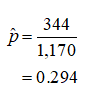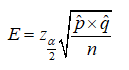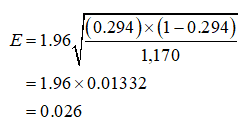# When the 2000 GSS asked subjects whether they would be willing to accept cuts in their standard of living to protect the environment, 344 of 1170 subjects said yes.Use 3 decimal places. (a) What is the estimate of the population proportion who would answer yes?(b) What is the margin of error for this estimate? (Assume a 95% confidence level.)(c) Find a 95% confidence interval for the population proportion.(d) Which of the following assumptions are needed for the interval in (c) to be valid?

Question
12 views

When the 2000 GSS asked subjects whether they would be willing to accept cuts in their standard of living to protect the environment, 344 of 1170 subjects said yes.

Use 3 decimal places. (a) What is the estimate of the population proportion who would answer yes?

(b) What is the margin of error for this estimate? (Assume a 95% confidence level.)

(c) Find a 95% confidence interval for the population proportion.
(d) Which of the following assumptions are needed for the interval in (c) to be valid?

check_circle

Step 1

Hi! Thank you for posting the question. Since your question has more than three sub-parts, we have solved the first three sub-parts for you. If you need help with any other parts, please re-post the question and mention the part you need help with.

Here, n=1,170 subjects and the number of respondents who said “yes” is 344.

a.

The estimate of the population proportion will be obtained as given below:Step 2

Margin of error (E):

The margin of error for proportion p is given by,b.

Computing the margin of error for population proportion:

The confidence level is 95%. Therefore, the significance level, α = 0.05 and the z value corresponding to α/2 is 1.96.  The margin of error for population proportion is given by:...

### Want to see the full answer?

See Solution

#### Want to see this answer and more?

Solutions are written by subject experts who are available 24/7. Questions are typically answered within 1 hour.*

See Solution
*Response times may vary by subject and question.
Tagged in